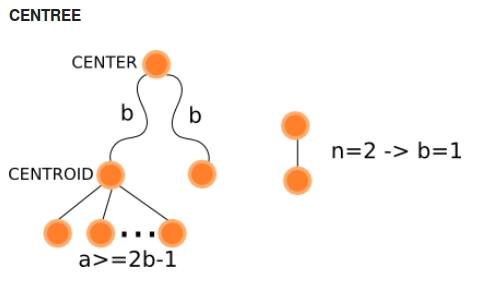# CENTREE - Editorial

Author: Alei Reyes
Primary Tester: Hussain Kara Fallah
Secondary Tester: Kacper Walentynowicz
Editorialist: Hussain Kara Fallah

Easy

Graph Theory

### PROBLEM:

Construct a tree of N nodes such that the maximum distance between one of its centers and one of its centroids is exactly B.

A vertex u is a centroid if after removing u from the tree, the size of any of the resulting connected components is at most n/2.

Let f(x) be the greatest distance from u to any other vertex of the tree. A vertex u is a center if f(u) ≤ f(v) for any other vertex v.

### EXPLANATION:

First of all let’s create a tree consisting of a single node numbered 1 and assuming that it will be our centroid.

Now we should construct a chain consisting of B edges ending in a node representing our future center and this node is numbered B+1. Currently our tree is composed of a chain containing B+1 nodes. Currently node B+1 is not the center of our tree. So to make it our center we should extend this chain from this node by B extra nodes. Resulting a chain consisting of 2*B+1 node where the ith node and the (i+1)th node are connected by an edge for each 0 < i < 2*B+1

Now we assured that node B+1 is our center. And clearly node 1 is not our centroid. So we must make use of the remaining nodes and attach each one of them directly to node 1 (Each of them connected to 1 by a directed edge so we keep our center unchanged). Of course we must have enough nodes to ensure that. Removing node 1 would leave us with a subtree consisting of 2*B nodes, and subtrees made of single nodes (we attached).

As a conclusion we can say that for any N,B such that :

2*B ≤ N/2 -> 4*B ≤ N

A solution exists and can be constructed by this way. For cases where 4*B > N, constructing such a tree is impossible.

Of course we must handle this special case :

Case n = 2:

if B = 1 the tree made of 2 nodes connected by an edge is the solution, for other values of B the answer is NO

This image briefly describes the configuration of our tree:### AUTHOR’S AND TESTER’S SOLUTIONS:

AUTHOR’s solution: [Here] 9
TESTER’s solution: [Here] 8

2 Likes

I am familiar with this problem. Try to connect with some professor or something like that. I know that it’s trivial, but one time i asked tutor and he helped me with my case studies

Can someone please help me with definition of center of the tree?? I am unable to figure out exactly is the center. I assumed its the node from which distance to any other node is least. But that didnt fit.

Can someone give explain to me the center of tree, and in light of the definition, explain-

``Currently node B+1 is not the center of our tree. So to make it our center we should extend this chain from this node by B extra nodes. Resulting a chain consisting of 2*B+1 node where the ith node and the (i+1)th node are connected by an edge for each 0 < i < 2*B+1``

I was damned on the n=2, b=0 case in which the answer must be “NO” and all other things were correct. Sad3 Likes

You can understand center of tree as:-

For each node find the largest distance from that node to any other node. So for each node you will get a number (largest distance from it).Now the node with the least of these numbers is the center.

In light to the above definition, it just states that for node B+1 to remain center, we have to extend the chain further from it as else maybe node B may become center and B+1 does not.

I don’t quite understand the n == 2 edge case. Why is the answer NO when b == 0? With an edge (1, 2), aren’t each of the two nodes both centroids and centres?

Yes, but B is defined as the maximum distance between a center and a centroid. Thus here it’s 1 not zero.

Thanks bro!! Got it nowAhh! The maximum distance! Of course…

Thank you for the directionWhy is the length of the largest chain considered to be 2B + 1 , and not 2(B+1)+1, as there will be an extra node which would be attached to the centroid.I mean, When the chain has to be extended from the centre at that time shouldn’t we be adding B+1 nodes instead of B, as there will be an extra node which will be attached to the Centroid.

After forming a chain of length 2B+1 then clearly node B+1 is the center of our tree. Attaching some nodes to our tree would make the diameter equal to 2B+2 so we have 2 centers. But we are taking the maximum distance between a center and a centroid (which is node 1 definitely)

Can someone explain me the definition of centeroid and center in simple words, i’m not getting it…!!!

Here
is the link to my editorial with little detailed explanation of centroid and center, i too faced quite a problem in understanding these definations. Hope it helps.

//